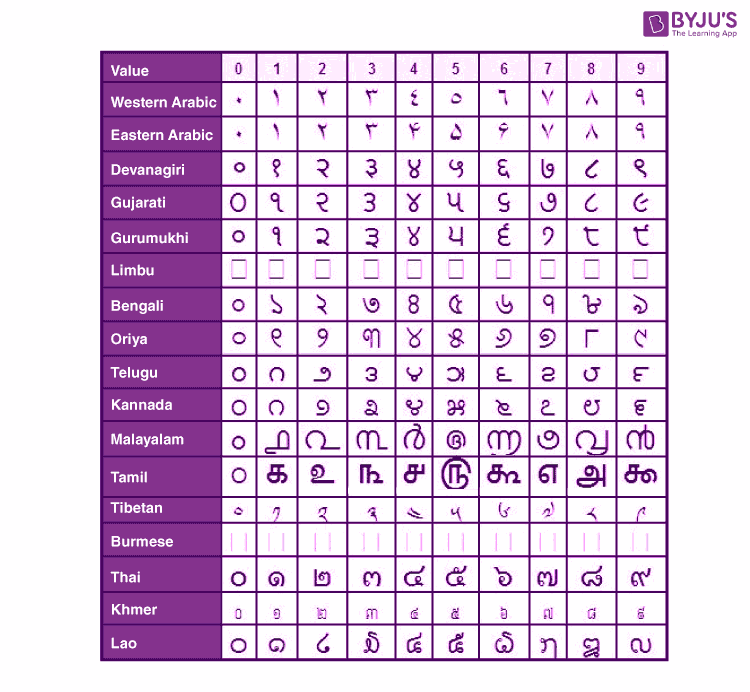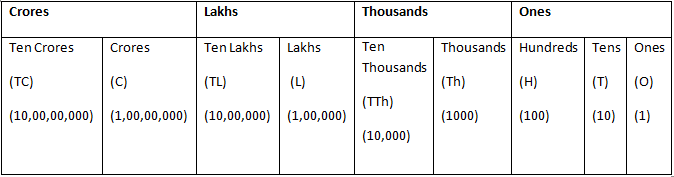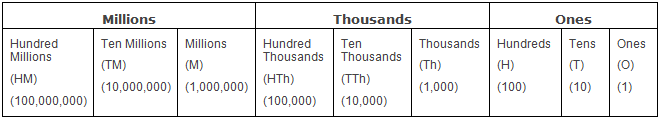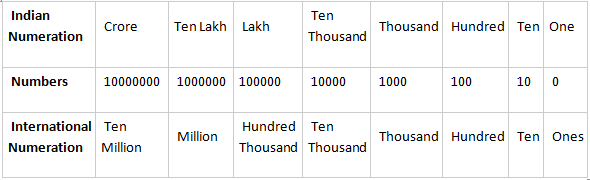# Numeral System: Indian And International

Long before the numbers were invented, counting was done using some kind of physical objects such as sticks or pebbles. Post that, lines and marks on rocks and pottery also continued for a long time for counting various things. Eventually, the numbers came into existence. Subsequently, then there was a need to adopt a standard system for counting, that is, a mathematical notation for expressing numbers, using digits or symbols. This is known as the numeral system or the system of numeration. Moreover, across different countries and periods, the numbers developed also differed. This is one reason we have a large number of numeral systems, e.g., Roman, Greek, Arabic, binary, decimal, etc. However, here we would confine our discussion to the Indian and international numeral system.

## What is Meant by the Numeral System?

In Mathematics, a numeral system is defined as a writing system to express the number in a consistent manner. The most commonly used numeral system is the Hindu-Arabic numeral system. It is originated in India, and now it is used throughout the world. It is considered as a  positional base 10 system. The positional value of each digit in a number is explained with the help of a place value chart.Hindu-Arabic Numeral System

Here, the positional values of Indian and International numeral systems are explained below.

## Indian Numeral System

Let us consider a number, say 225. Notice that the digit 2 is used twice in this number. Both of them have a different value. We differentiate them by stating their place value, which is defined as the numerical value of a digit on the basis of its position in a number. So, the place value of the leftmost 2 is Hundreds while the one in the center is Tens.

Coming back to the Indian numeral system, the place values of digits go in the sequence of Ones, Tens, Hundreds, Thousands, Ten Thousand, Lakhs, Ten Lakhs, Crores and so on.

In the number 10,23,45,678 the place values of each digit are:

• 8 – Ones
• 7 – Tens
• 6 – Hundreds
• 5 – Thousands
• 4 – Ten Thousand
• 3 – Lakhs
• 2 – Ten Lakhs
• 0 – Crores
• 1 – Ten Crores

The relationship between them is:

• 1 hundred = 10 tens
• 1 thousand = 10 hundreds = 100 tens
• 1 lakh = 100 thousands = 1000 hundreds
• 1 crore = 100 lakhs = 10,000 thousandsIndian Numeral System

### How Many Zeros in 1 Crore?

There are 7 zeros in 1 crore.

We know, 1 crore = 100 lakhs, and 1 lakh is equivalent to 1,00,000

As there are 5 zeros in lakhs.

1 crore can be written as

1 crore = 100 (100000)

1 crore = 1, 00,00,000.

Thus, the number of zeros in 1 crore is 7.

## International Numeral System

The place values of digits go in the sequence of Ones, Tens, Hundreds, Thousands, Ten Thousand, Hundred Thousands, Millions, Ten Million and so on, in the international numeral system. In the number 12,345,678 the place values of each digit are:

• 8 – Ones
• 7 – Tens
• 6 – Hundreds
• 5 – Thousands
• 4 – Ten Thousand
• 3 – Hundred Thousands
• 2 – Millions
• 1 – Ten Million

The relations between them are:

• 1 hundred = 10 tens
• 1 thousand = 10 hundreds = 100 tens
• 1 million = 1000 thousand
• 1 billion = 1000 millionsInternational Numeral System

### How Many Zeros in a Million?

There are 6 zeros in a million. (i.e., 1 million = 1, 000, 000)

We can say 1 million = 1000 thousand.

We know that, 1 thousand = 1000.

As there are 3 zeros in a thousand,

1 million is written as

1 million = 1000 (100)

1 million = 1, 00, 0000

Hence, the number of zeros in a million is 6.

### Comparison Between Indian and International Numeral System

Comparing the two numeral systems we observe that:

• 100 thousand = 1 lakh
• 1 million = 10 lakhs
• 10 millions = 1 crore
• 100 millions= 10 crores### Placement of Comma

Commas are placed in large numbers to help us read and write them easily. In Indian and international systems, the commas are placed at different positions.

As per the Indian numeral system, the first comma is placed after the hundreds place post which they are placed after every two digits. E.g., 1,23,45,67,890

As per the international numeral system, the first comma is placed after the hundreds place, post which they are placed after every three digits. E.g., 1,234,567,890

## Frequently Asked Questions on Numeral System

### What is meant by place value?

In Maths, the place value of any digit in a number is defined by its position. For example, 345 is a number, The place value of 4 is 40 because 4 is in ten’s position.

### What is the difference between the place value and face value?

The place value of a digit is determined by the position of the digit in a number, whereas the face value of a digit is the digit itself. For example, 297 is a number. The place value of 2 is 200, whereas the face value of 2 is 2.

### What is the difference between the Indian and International numeral system?

The main difference between the Indian and International numeral system is the placement of commas (separator). In the Indian system, lakhs are written after thousands, whereas in the International system, millions are written after thousands.

### Write down the comparison between the Indian and International numeral systems?

The comparison between Indian and International numeral systems are:
100 thousand = 1 lakh
1 million = 10 lakhs
10 millions = 1 crore
100 millions = 10 crores

### How many zeros are in 10 lakhs?

There are 6 zeros in 10 lakhs.
We know that 1 lakh = 1,00, 000
Thus, 10 lakhs = 10 x 1, 00, 000
10 lakhs = 10, 00, 000.

Quiz on Numeral system

1. Thanks so much for the enlightenment

2. vijay pratap

3. Vinit Attarde

Hi I have doubt

4. Christ Akanksha kerketta

It a nice app